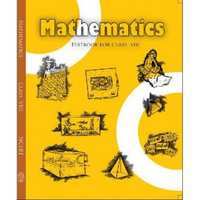# NCERT solutions for Class 8 Maths Textbook chapter 16 - Playing with Numbers [Latest edition]

#### Chapters## Chapter 16: Playing with Numbers

Exercise 16.1Exercise 16.2
Exercise 16.1 [Pages 255 - 256]

### NCERT solutions for Class 8 Maths Textbook Chapter 16 Playing with Numbers Exercise 16.1 [Pages 255 - 256]

Exercise 16.1 | Q 1 | Page 255

Find the values of the letters in each of the following and give reasons for the steps involved.

3 A

+  2 5

-------

B 2

-------

Exercise 16.1 | Q 2 | Page 255

Find the values of the letters in the following and give reasons for the steps involved.

4 A

+ 9 8

------

C  B 3

------

Exercise 16.1 | Q 3 | Page 255

Find the value of the letter in the following and give reasons for the steps involved.

1 A

x A

------

9 A

------

Exercise 16.1 | Q 4 | Page 256

Find the values of the letters in the following and give reasons for the steps involved.

A B

+ 3 7

------

6 A

------

Exercise 16.1 | Q 5 | Page 256

Find the values of the letters in the following and give reasons for the steps involved

A  B

x  3

-------

C  A  B

-------

Exercise 16.1 | Q 6 | Page 256

Find the values of the letters in the following and give reasons for the steps involved.

A  B

x  5

-------

C  A B

-------

Exercise 16.1 | Q 7 | Page 256

Find the values of the letters in the following and give reasons for the steps involved.

A  B

x   6

-------

B  B  B

-------

Exercise 16.1 | Q 8 | Page 256

Find the values of the letters in the following and give reasons for the steps involved.

A  1

+ 1  B

-------

B  0

-------

Exercise 16.1 | Q 9 | Page 256

Find the values of the letters in the following and give reasons for the steps involved.

2  A  B

+ A  B  1

---------

B  1  8

----------

Exercise 16.1 | Q 10 | Page 256

Find the values of the letters in the following and give reasons for the steps involved.

1  2  A

+ 6  A  B

---------

A  0  9

---------

Exercise 16.2 [Page 260]

### NCERT solutions for Class 8 Maths Textbook Chapter 16 Playing with Numbers Exercise 16.2 [Page 260]

Exercise 16.2 | Q 1 | Page 260

If 21y5 is a multiple of 9, where y is a digit, what is the value of y?

Exercise 16.2 | Q 2 | Page 260

If 31z5 is a multiple of 9, where z is a digit, what is the value of z? You will find that there are two answers for the last problem. Why is this so?

Exercise 16.2 | Q 3 | Page 260

If 24x is a multiple of 3, where x is a digit, what is the value of x?

(Since 24x is a multiple of 3, its sum of digits 6 + x is a multiple of 3; so 6 + x is one of these numbers: 0, 3, 6, 9, 12, 15, 18…. But since x is a digit, it can only be that 6 + x = 6 or 9 or 12 or 15. Therefore, x = 0 or 3 or 6 or 9. Thus, x can have any of four different values)

Exercise 16.2 | Q 4 | Page 260

If 31z5 is a multiple of 3, where z is a digit, what might be the values of z?

## Chapter 16: Playing with Numbers

Exercise 16.1Exercise 16.2## NCERT solutions for Class 8 Maths Textbook chapter 16 - Playing with Numbers

NCERT solutions for Class 8 Maths Textbook chapter 16 (Playing with Numbers) include all questions with solution and detail explanation. This will clear students doubts about any question and improve application skills while preparing for board exams. The detailed, step-by-step solutions will help you understand the concepts better and clear your confusions, if any. Shaalaa.com has the CBSE Class 8 Maths Textbook solutions in a manner that help students grasp basic concepts better and faster.

Further, we at Shaalaa.com provide such solutions so that students can prepare for written exams. NCERT textbook solutions can be a core help for self-study and acts as a perfect self-help guidance for students.

Concepts covered in Class 8 Maths Textbook chapter 16 Playing with Numbers are Divisibility of 5, Numbers in General Form, Games with Numbers, Letters for Digits, Divisibility by 2, Divisibility by 3, Divisibility of 10, Divisibility by 9.

Using NCERT Class 8 solutions Playing with Numbers exercise by students are an easy way to prepare for the exams, as they involve solutions arranged chapter-wise also page wise. The questions involved in NCERT Solutions are important questions that can be asked in the final exam. Maximum students of CBSE Class 8 prefer NCERT Textbook Solutions to score more in exam.

Get the free view of chapter 16 Playing with Numbers Class 8 extra questions for Class 8 Maths Textbook and can use Shaalaa.com to keep it handy for your exam preparation